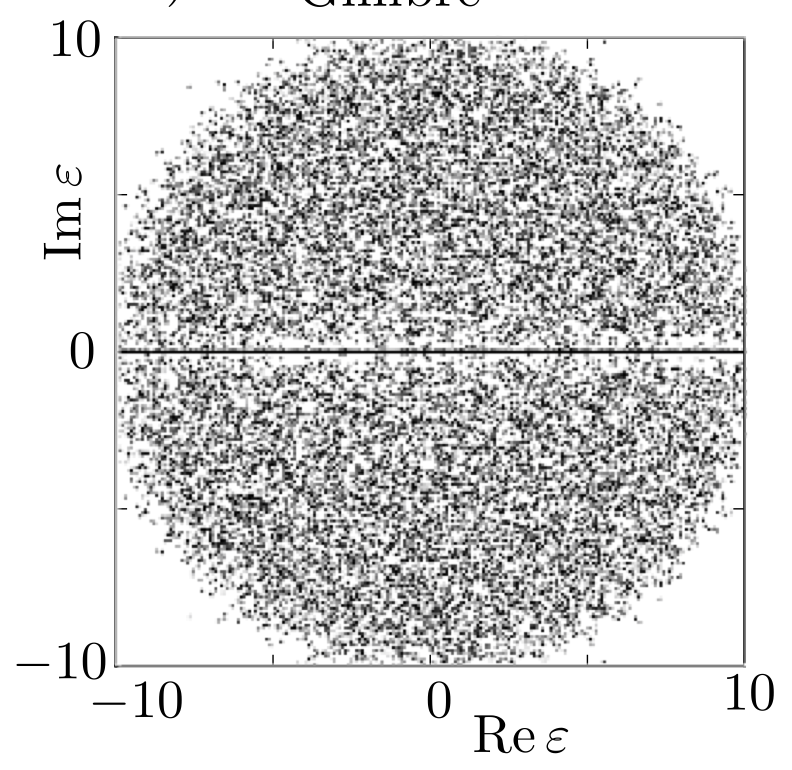# Eigenvalues and eigenvectors of Gaussian random matrices

Let us assume we have a square matrix $$A$$ whose entries are sampled from a standard Gaussian distribution of mean $$0$$. Do we have any information about the distribution of its eigenvalues?

Particularly, I'm aware that there are different results on symmetric gaussian matrices (or, the Gaussian orthogonal ensemble of $$A$$):

• The eigenvalues follow a semicircle law

Is there an equivalent result for standard, non-symmetric Gaussian matrices?

• I have answered this for the ensemble of nonsymmetric matrices, but do note that your statements on the symmetric case (GOE) are not correct: the eigenvalues follow a semicircle law, not a Gaussian distribution. Mar 28, 2020 at 11:42
• You are right. Thanks for the answer, I'll edit for future readers! Mar 30, 2020 at 17:22

This is the Ginibre ensemble, see Eigenvalue statistics of the real Ginibre ensemble for the eigenvalue distribution. For an $$N\times N$$ matrix with $$N\gg 1$$ there are on average $$\sqrt{2N/\pi}$$ eigenvalues on the real axis, uniformly in the interval $$(-\sqrt N,\sqrt N$$). The rest of the eigenvalues fill a disc of radius $$\sqrt N$$ in the complex plane, uniformly except for a depleted strip along the real axis. Here is a scatter plot of the eigenvalues for $$N=100$$ (taken from arXiv:1305.2924).